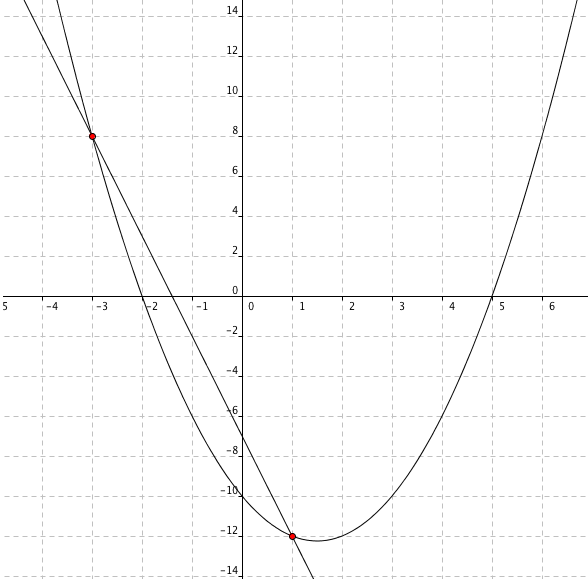### Home > AC > Chapter 12 > Lesson 12.1.3 > Problem12-30

12-30.

Graph the two functions below and find all points where they intersect. List all points in the form $(x, y)$.

$f\left(x\right)=x^2-3x-10$
$g\left(x\right)=-5x-7$What are the two intersecting points?